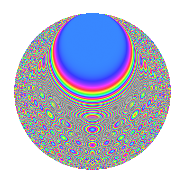# Properties

 Label 128.2.kLevel $128$ Weight $2$ Character orbit 128.k Rep. character $\chi_{128}(5,\cdot)$ Character field $\Q(\zeta_{32})$ Dimension $240$ Newform subspaces $1$ Sturm bound $32$ Trace bound $0$

# Related objects

## Defining parameters

 Level: $$N$$ $$=$$ $$128 = 2^{7}$$ Weight: $$k$$ $$=$$ $$2$$ Character orbit: $$[\chi]$$ $$=$$ 128.k (of order $$32$$ and degree $$16$$) Character conductor: $$\operatorname{cond}(\chi)$$ $$=$$ $$128$$ Character field: $$\Q(\zeta_{32})$$ Newform subspaces: $$1$$ Sturm bound: $$32$$ Trace bound: $$0$$

## Dimensions

The following table gives the dimensions of various subspaces of $$M_{2}(128, [\chi])$$.

Total New Old
Modular forms 272 272 0
Cusp forms 240 240 0
Eisenstein series 32 32 0

## Trace form

 $$240q - 16q^{2} - 16q^{3} - 16q^{4} - 16q^{5} - 16q^{6} - 16q^{7} - 16q^{8} - 16q^{9} + O(q^{10})$$ $$240q - 16q^{2} - 16q^{3} - 16q^{4} - 16q^{5} - 16q^{6} - 16q^{7} - 16q^{8} - 16q^{9} - 16q^{10} - 16q^{11} - 16q^{12} - 16q^{13} - 16q^{14} - 16q^{15} - 16q^{16} - 16q^{17} - 16q^{18} - 16q^{19} - 16q^{20} - 16q^{21} - 16q^{22} - 16q^{23} - 16q^{24} - 16q^{25} - 16q^{26} - 16q^{27} - 16q^{28} - 16q^{29} - 16q^{30} - 16q^{31} - 16q^{32} - 16q^{33} - 16q^{34} - 16q^{35} - 16q^{36} - 16q^{37} - 16q^{38} - 16q^{39} - 16q^{40} - 16q^{41} - 16q^{42} - 16q^{43} - 16q^{44} - 16q^{45} - 16q^{46} - 16q^{47} - 16q^{48} - 16q^{49} + 32q^{50} - 16q^{51} + 80q^{52} - 16q^{53} + 112q^{54} - 16q^{55} + 96q^{56} - 16q^{57} + 128q^{58} - 16q^{59} + 176q^{60} - 16q^{61} + 80q^{62} + 176q^{64} + 176q^{66} - 16q^{67} + 80q^{68} - 16q^{69} + 176q^{70} - 16q^{71} + 128q^{72} - 16q^{73} + 96q^{74} - 16q^{75} + 112q^{76} - 16q^{77} + 80q^{78} - 16q^{79} + 32q^{80} - 16q^{81} - 16q^{82} - 16q^{83} - 16q^{84} - 16q^{85} - 16q^{86} - 16q^{87} - 16q^{88} - 16q^{89} - 16q^{90} - 16q^{91} - 16q^{92} - 16q^{93} - 16q^{94} - 16q^{95} - 16q^{96} - 16q^{97} - 16q^{98} - 16q^{99} + O(q^{100})$$

## Decomposition of $$S_{2}^{\mathrm{new}}(128, [\chi])$$ into newform subspaces

Label Dim. $$A$$ Field CM Traces $q$-expansion
$$a_2$$ $$a_3$$ $$a_5$$ $$a_7$$
128.2.k.a $$240$$ $$1.022$$ None $$-16$$ $$-16$$ $$-16$$ $$-16$$